Question

# 1. Multiple choice. Circle all the correct answers a) You flip a coin 100,000 times and...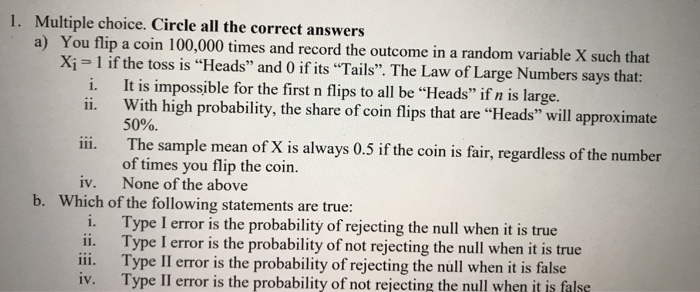1. Multiple choice. Circle all the correct answers a) You flip a coin 100,000 times and record the outcome in a Xi 1 if the toss is "Heads" and 0 if its "Tails. The Law of Large Numbers says that: i. ii. It is impossible for the first n flips to all be "Heads" if n is large. With high probability, the share of coin flips that are "Heads" will approximate 50%. The sample mean of X is always 0.5 if the coin is fair, regardless of the number of times you flip the coin. None of the above iv. Which of the following statements are true: b. i. Type I error is the probability of rejecting the null when it is true ii. Type I error is the probability of not rejecting the null when it is true i. Type II error is the probability of rejecting the null when it is false iv. Type II error is the probability of not rejecting the null when it is false

a) LAW OF LARGE NUMBERS say that if an experiment is repeated independently by large number of times, then sample mean approaches theoretical mean. So, (iii) cannot be accurate.

(ii) is accurate from if we go by definition mentioned above. (i) cannot be true is n is large as the distribution becomes normal. For all first n flips to be head, the distribution is poisson distribution. But, LLN states that when n is large all distribution tend to be normal distribution.

Thus, (ii) is the only CORRECT answer.

b) (i) and (iv) are the statements that are CORRECT. For clearer understanding , we use the following example.

Consider the null hypothesis (Ho) that a person is telling truth. Thus alternative hypothesis (Ha) is the person is lying.

The court gives a verdict of either "GUILTY" or "NOT GUILTY".

HO HA
NOT GUILTY RIGHT TYPE I ERROR (FALSE POSITIVE)
GUILTY TYPE II ERROR (FALSE NEGATIVE) RIGHT

If the person is telling truth and court says " NOT GUILTY" then NO ERROR.

If the person is lying and court says "GUILTY" then NO ERROR.

If the person is telling truth and court says " GUILTY" then TYPE II ERROR or FALSE NEGATIVE as court failed to REJECT FALSE NULL HYPOTHESIS.

If the person is lying and court says "NOT GUILTY" then TYPE I ERROR or FALSE POSITIVE as court REJECTED TRUE NULL HYPOTHESIS.

#### Earn Coins

Coins can be redeemed for fabulous gifts.

Similar Homework Help Questions
• ### Question 2 Suppose you have a fair coin (a coin is considered fair if there is...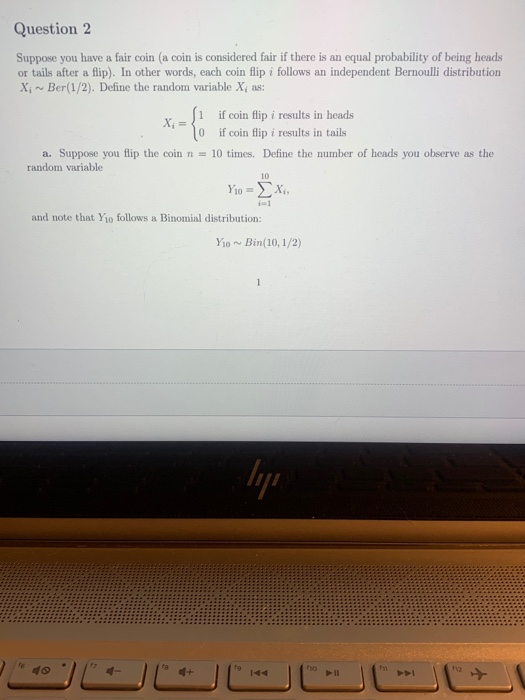Question 2 Suppose you have a fair coin (a coin is considered fair if there is an equal probability of being heads or tails after a flip). In other words, each coin flip i follows an independent Bernoulli distribution X Ber(1/2). Define the random variable X, as: i if coin flip i results in heads 10 if coin flip i results in tails a. Suppose you flip the coin n = 10 times. Define the number of heads you observe...

• ### You flip the same coin 90 mores times (100 total flips). If half of the 90...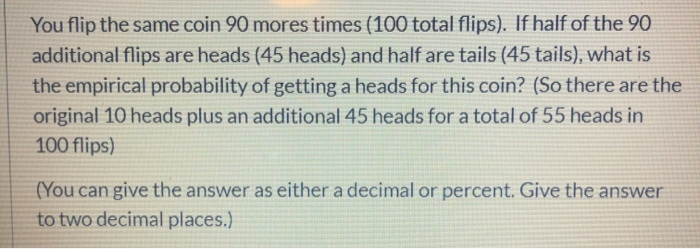You flip the same coin 90 mores times (100 total flips). If half of the 90 additional flips are heads (45 heads) and half are tails (45 tails), what is the empirical probability of getting a heads for this coin? (So there are the original 10 heads plus an additional 45 heads for a total of 55 heads in 100 flips) (You can give the answer as either a decimal or percent. Give the answer to two decimal places.)

• ### Exercise 1.16. We flip a fair coin five times

Exercise 1.16. We flip a fair coin five times. For every heads you pay me \$1 and for every tails I pay you \$1. Let X denote my net winnings at the end of five flips. Find the possible values and the probability mass function of X.

• ### Suppose we flip a fair coin n times. We say that the sequence is balanced when there are equal number of heads and tails...

Suppose we flip a fair coin n times. We say that the sequence is balanced when there are equal number of heads and tails. For example, if we flip the coin 10 times and the results are HT HHT HT T HH, then this sequence balanced 2 times, i.e. at position 2 and position 8 (after the second and eighth flips). In terms of n, what is the expected number of times the sequence is balanced within n flips?

• ### You toss a penny and observe whether it lands heads up or tails up. Suppose the...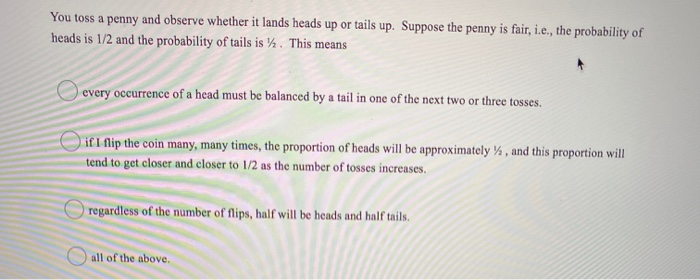You toss a penny and observe whether it lands heads up or tails up. Suppose the penny is fair, i.e., the probability of heads is 1/2 and the probability of tails is y. This means every occurrence of a head must be balanced by a tail in one of the next two or three tosses. if I flip the coin many, many times, the proportion of heads will be approximately %, and this proportion will tend to get closer and...

• ### Coin Toss Program

I have this problem to write in C++ class"Write a program that has a function prototype before main and an implementation of the function after main. The function to be implemented is a coin tosssimulation using the random number generator. The function should return 1 or true 50% of the time and 0 or false 50% of the time. Use srand and the system time tomake the program run differently each time. (srand(time(NULL));). Keep track of the number of head...

• ### In C++ please Create a coin-flipping game. Ask the user how many times to flip the...

In C++ please Create a coin-flipping game. Ask the user how many times to flip the coin, and use the random function to determine heads or tails each time a coin is flipped. Assume the user starts with \$50. Every time the coin is flipped calculate the total (heads +\$10, tails -\$10). Create another function to test if the user has gone broke yet (THIS FUNCTION MUST RETURN A BOOLEAN TRUE/FALSE VALUE). End the program when the user is broke...

• ### PLEASE ANSWER QUESTION # 5 a and b !!!!!!!!!! 4) Chapter 4-5: a) You flip a balanced coin 3 times. What is the probabil...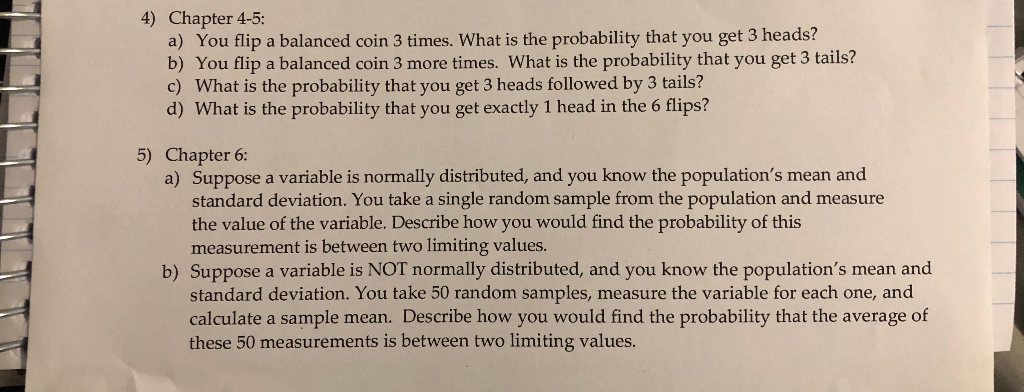PLEASE ANSWER QUESTION # 5 a and b !!!!!!!!!! 4) Chapter 4-5: a) You flip a balanced coin 3 times. What is the probability that you get 3 heads? b) You flip a balanced coin 3 more times. What is the probability that you get 3 tails? c) What is the probability that you get 3 heads followed by 3 tails? d) What is the probability that you get exactly 1 head in the 6 flips? 5) Chapter 6: a)...

• ### 3 Probability and Statistics [10 pts] Consider a sample of data S obtained by flipping a...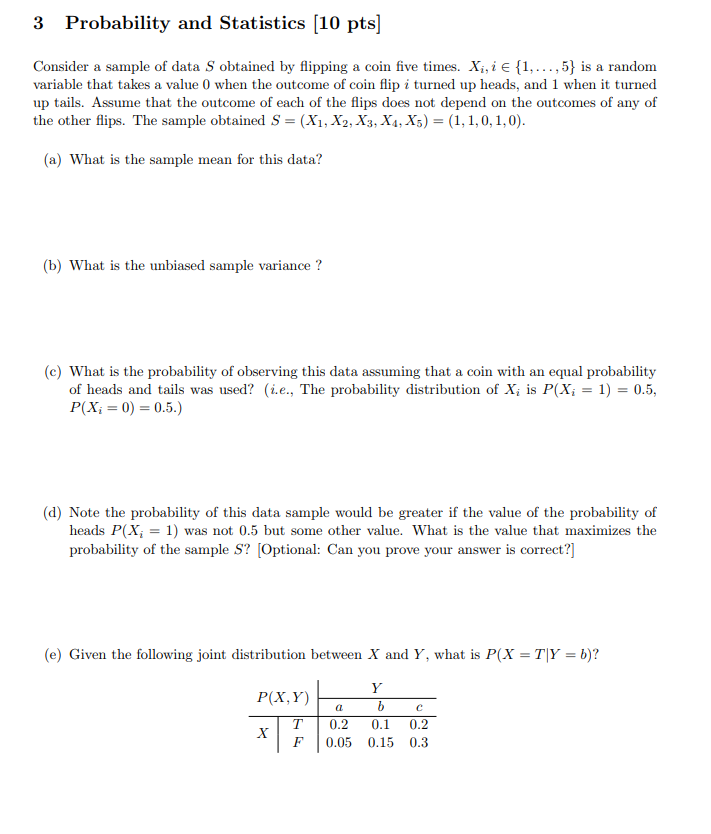3 Probability and Statistics [10 pts] Consider a sample of data S obtained by flipping a coin five times. X,,i e..,5) is a random variable that takes a value 0 when the outcome of coin flip i turned up heads, and 1 when it turned up tails. Assume that the outcome of each of the flips does not depend on the outcomes of any of the other flips. The sample obtained S - (Xi, X2,X3, X, Xs) (1, 1,0,1,0 (a)...